Courses

Short Answer Type Questions: Surface Areas & Volumes Notes | Study Mathematics (Maths) Class 9 - Class 9

Class 9: Short Answer Type Questions: Surface Areas & Volumes Notes | Study Mathematics (Maths) Class 9 - Class 9

The document Short Answer Type Questions: Surface Areas & Volumes Notes | Study Mathematics (Maths) Class 9 - Class 9 is a part of the Class 9 Course Mathematics (Maths) Class 9.
All you need of Class 9 at this link: Class 9

Question 1. The length, breadth and height of a room are 4 m, 3 m and 3 m respectively. Find the lateral surface area of the room.
Solution:
Here, l = 4 m, b = 3 m and h = 3 m.
Lateral surface area = Area of four walls = 2(l + b)h = 2(4 + 3) x 3 m2 = 2 x 7 x 3 m= 42 m2
∴ The required lateral surface of the room is 42 m2.

Question 2. The floor area of a room is 100 m2 and its height is 8 m. Find its volume.
Solution:
∵ Volume of a cuboid = [Base area] x Height
∴ Volume of the room = [Area of the floor] x height = 100 m2 x 8 m = 800 m3
Thus, the volume of the room = 800 m3.

Question 3. If the total surface area of a cube is 216 cm2, then find its volume.
Solution:
Let each side of the cube be x.
∴ Total surface area = 6x2
⇒ 6x= 216
⇒   x2 =(216/6) = 36
⇒ x = √36 = 6 cm

∴ Volume = (side)3 = 63 = 6 x 6 x 6
= 216 cm3

Question 4. If the circumference of the base of a right circular cylinder is 110 cm, then find its base area.
Solution:
Let r be the radius of the base of the cylinder.

∴ Circumference = 2πr = 2 x(22/7)x r
Now, 2 x(22/7) x r= 110

⇒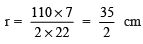Now, Base area =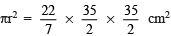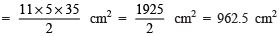Question 5. The curved surface area of a cylinder is 4400 cm2. If the circumference of its base is 110 cm, then find its height.
Solution:
Curved surface area of the cylinder = 2πrh
Circumference = 2πr

∴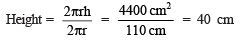Thus, the height of the cylinder is 40 cm.

Question 6. The radii of two cylinders are in the ratio of 2 : 3 and heights are in the ratio of 5 : 3.
Find the ratio of their volumes.
Solution:
Ratio of the radii = 2 : 3 Let the radii be 2r and 3r Also, their heights are in the ratio of 5:3 Let the height be 5h and 3h
∴ Ratio of their volumes =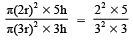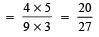Question 7. If the radius of a sphere is doubled, then find the ratio of their volumes.
Solution:
Let the radius of the original sphere = r
∴ Radius of new sphere = 2r

∴  Ratio of their volumes =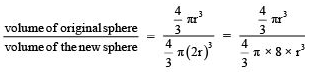Question 8. If the radius of a sphere is such that πr2 = 6cm2 then find its total surface area.
Solution:
∵ πr2 = 6cm
∴ Curved S.A. of the hemisphere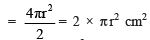= 2 × 6 cm2 = 12 cm
Also, plane S.A. of the hemisphere = π r2 = 6 cm
⇒ Total S.A. = C.S.A. + plane S.A. = 12 cm2 + 6 cm2 = 18 cm2

Question 1. The surface area of a sphere of radius 5 cm is five times the area of the curved surface of a cone of radius 4 cm. Find the height and the volume of the cone (taking π = (22/7).
Solution:
Surface area of the sphere = 4πr2 = 4 × π × 5 × 5 cm2
Curved surface area of the cone (with slant height as ‘ℓ’) = πrℓ = π × 4 × ℓ cm2

Since,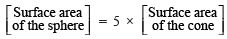∴ 4 × π × 5 × 5 = 5 × π × 4 × ℓ
⇒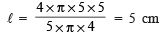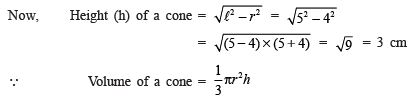∴ Volume of the cone =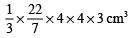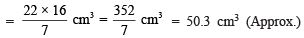Question 2. The radius of a sphere is increased by 10%. πrove that the volume will be increased by 33.1% apπroximately.
Solution:
The volume of a sphere =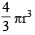Increased radius =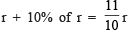∴ Increased volume =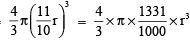= (4/3) x π x 1.331r3

Thus, Increase in volume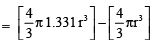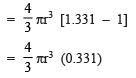∴ Percentage increase in volume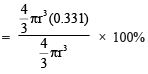= 0.331 × 100%
= 33.1%.

Question 3. Find the slant height of a cone whose radius is 7 cm and height is 24 cm.
Solution:
Here, h = 24 cm and r = 7 cm

Since,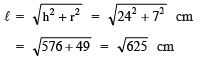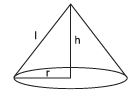= 25 cm
∴ Slant height = 25 cm.

Question 4. The radius of a cylinder is 7 cm. If its volume is 2002 cm3, then find its height and total surface area.
Solution:
Here, radius (r) = 7 cm
∴ Volume of the cylinder = = πr2h = (22/7) x 7 x 7 x h
Now,(22/7)x 7 x 7 x h = 2002

∴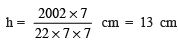Now, total surface area of the cylinder = 2πr2 + 2πrh = 2πr(r + h)
= 2 x(22/7) x 7 x (7 + 13) cm
= 44 × 20 cm2 = 880 cm

Question 5. The diameter of a road roller, 120 cm long is 84 cm. If it takes 500 complete revolutions to level a playground, find the cost of levelling it at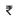2 per square metre.
Solution:
Here, radius (r) = 42 cm
Length of the roller = Height of the cylinder
⇒ h = 120 cm
∴ Curved surface area of the roller = 2πrh =2 x(22/7) x 42 x 120 cm
= 2 x 22 x 6 x 120 cm2 = 31680 cm2
∴ Area levelled in one revolution = 31680 cm2

⇒ Area levelled in 500 revolutions = 31680 x 500 cm2∴ Cost of levelling the playground =2 x 15840 =31680.

Question 6. A conical tent of radius 7 m and height 24 m is to be made. Find the cost of the 5 m wide cloth required at the rate of50 per metre.
Solution:
Radius of the base of the tent (r) = 7 m
Height (h) = 24 m
Slant height (ℓ)=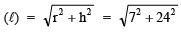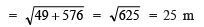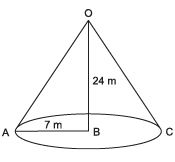Now, curved surface area of the conical tent = πrℓ
= (22/7) x 7 x 25 m2 = 22 x 25 m2 = 550 m
Let ‘ℓ’ be the length of the cloth.

∴ ℓ  x b = 550
⇒ ℓ  x 5 = 550
⇒ ℓ = (550/5) m = 110 m
∴ Cost of the cloth =50 x 110 =5500.

Solution:

∴ Volume of a lead ball =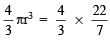x 1 x 1 x 1 cm3

= (4/3) x (22/7) cm3
Radius of the sphere (r) = 8 cm

∴ Volume of a sphere =x 8 x 8 x 8 cm3

Let the required number of balls = n
∴ [Volume of n-lead balls] = [Volume of the sphere]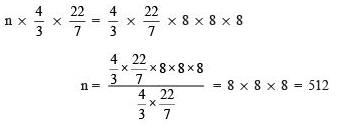Thus, the required number of balls is 512.

The document Short Answer Type Questions: Surface Areas & Volumes Notes | Study Mathematics (Maths) Class 9 - Class 9 is a part of the Class 9 Course Mathematics (Maths) Class 9.
All you need of Class 9 at this link: Class 9Use Code STAYHOME200 and get INR 200 additional OFF Use Coupon Code

Top Courses for Class 9Mathematics (Maths) Class 9

46 videos|351 docs|110 tests

Top Courses for Class 9Track your progress, build streaks, highlight & save important lessons and more!

,

,

,

,

,

,

,

,

,

,

,

,

,

,

,

,

,

,

,

,

,

;